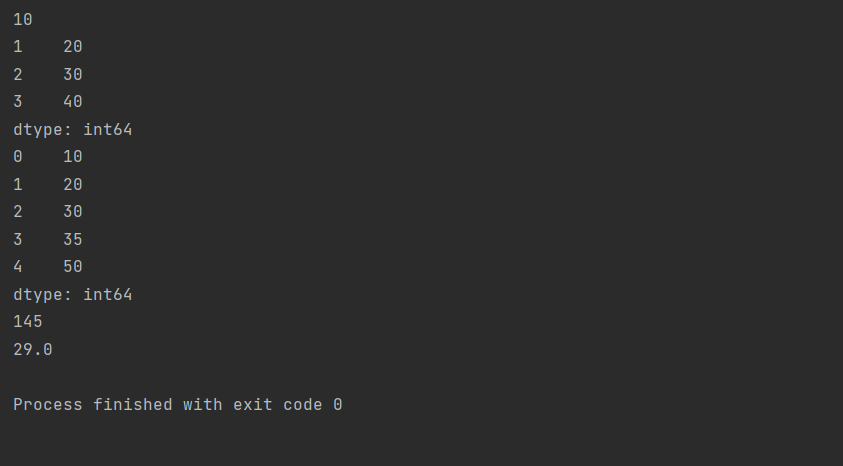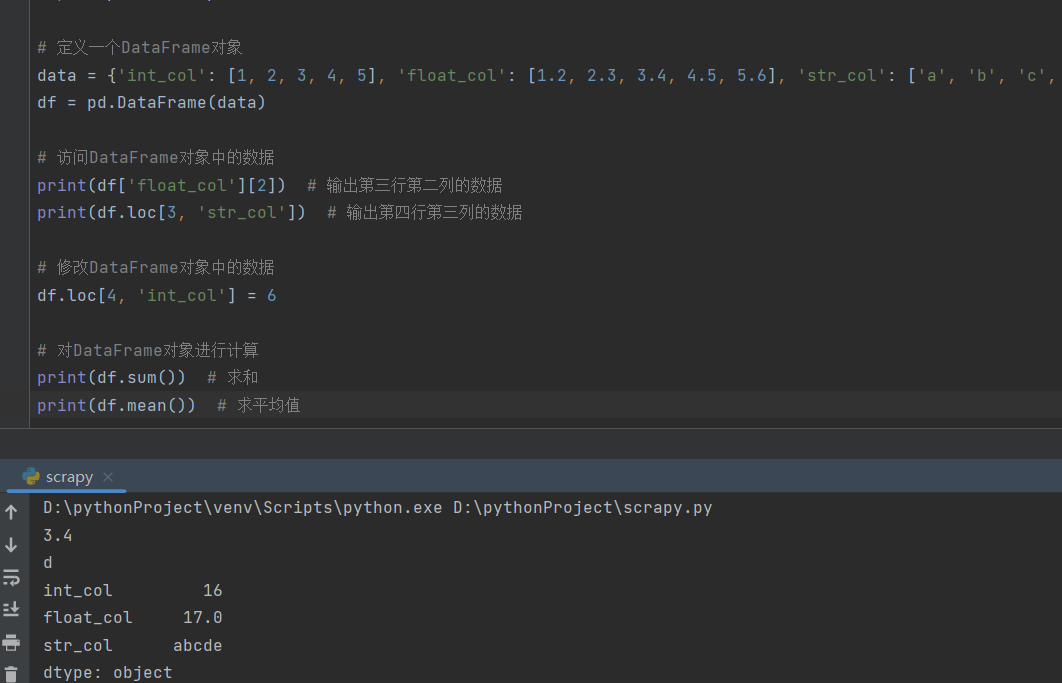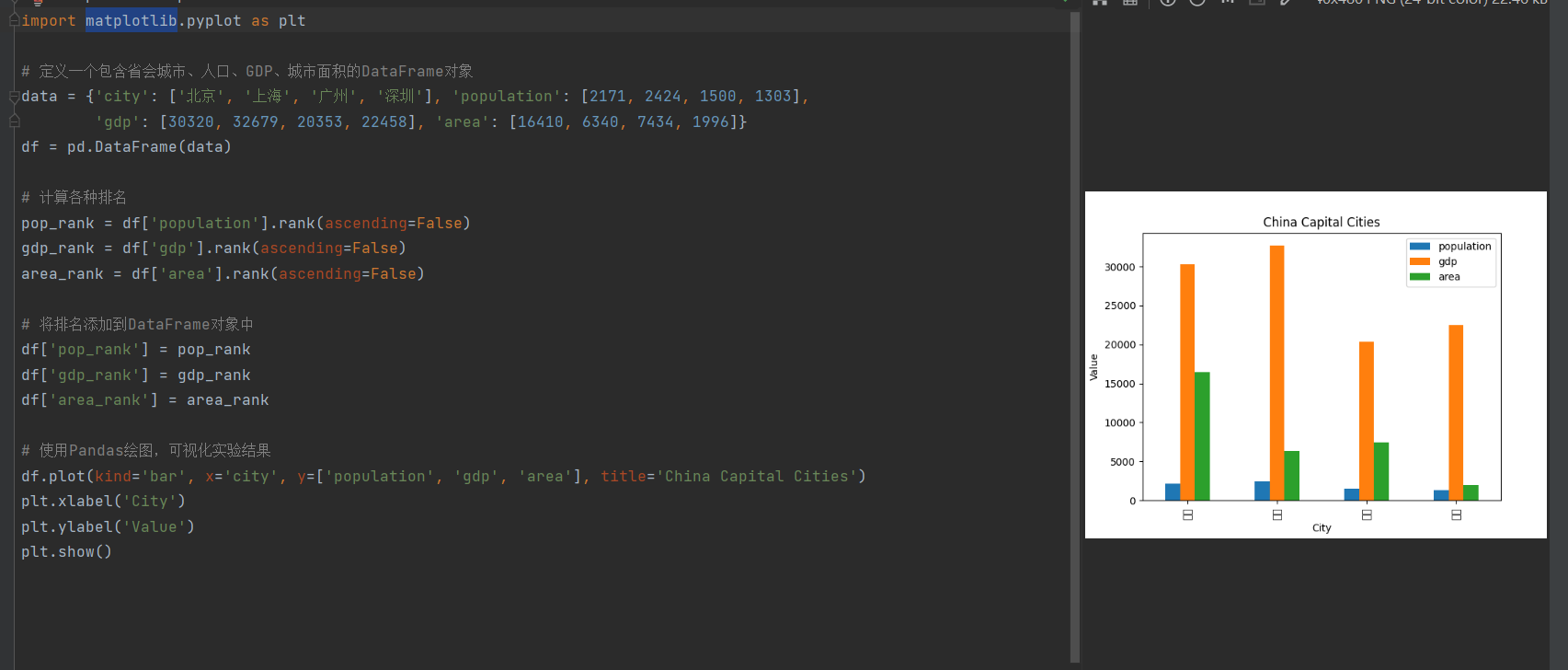# 2023（春）Python程序设计作业5：Pandas基础技能及综合应用

## 实验一：Series对象的应用

### 实验要求：

• 定义一个Series对象，包含5个整数数据；
• 访问、修改Series对象中的数据；
• 打印Series对象；
• 对Series对象进行计算，如求和、求平均值等。
• ### 修改代码：

• ``````import pandas as pd

# 定义一个Series对象
s = pd.Series([10, 20, 30, 40, 50])

# 访问Series对象中的数据
print(s)    # 输出第一个元素
print(s[1:4])  # 输出第2个到第4个元素

# 修改Series对象中的数据
s = 35

# 打印Series对象
print(s)

# 对Series对象进行计算
print(s.sum())  # 求和
print(s.mean())  # 求平均值
``````

修改的意图：

• 将访问的数据范围修改为索引1到索引4的元素（第2个到第4个元素）。
• 将索引3的元素修改为35。
• 代码运行的结果：
•结论：

通过修改代码，改变了访问的数据范围并修改了特定元素的值，再对Series对象进行了求和和求平均值的计算。根据运行结果，修改后的代码能够正确地实现，并得到了相应的结果。

## 实验二：DataFrame对象的应用

### 实验要求：

• 定义一个DataFrame对象，包含3个列，每列分别为整数、浮点数和字符串类型；
• 访问、修改DataFrame对象中的数据；
• 对DataFrame对象进行计算，如求和、求平均值等。
• 实验二：DataFrame对象的应用

要求：

• 定义一个DataFrame对象，包含3个列，每列分别为整数、浮点数和字符串类型；
• 访问、修改DataFrame对象中的数据；
• 对DataFrame对象进行计算，如求和、求平均值等
• 修改的代码：
• ``````import pandas as pd

# 定义一个DataFrame对象
data = {'int_col': [1, 2, 3, 4, 5], 'float_col': [1.2, 2.3, 3.4, 4.5, 5.6], 'str_col': ['a', 'b', 'c', 'd', 'e']}
df = pd.DataFrame(data)

# 访问DataFrame对象中的数据
print(df['float_col'])  # 输出第三行第二列的数据
print(df.loc[3, 'str_col'])  # 输出第四行第三列的数据

# 修改DataFrame对象中的数据
df.loc[4, 'int_col'] = 6

# 对DataFrame对象进行计算
print(df.sum())  # 求和
print(df.mean())  # 求平均值
``````

修改的意图

• 将访问的数据修改为第三行第二列的元素和第四行第三列的元素。
• 将第五行第一列的元素修改为6。

运行结果：我们改变了访问的数据和特定位置的元素的值。最后，我们对DataFrame对象进行了求和和求平均值的计算。根据运行结果，修改后的代码能够正确地实现我们的意图，并得到了相应的结果。

## 实验三：综合实例

### 实验要求：

• 定义一个包含省会城市、人口、GDP、城市面积的DataFrame对象；
• 计算各种排名，如人口最多的城市、GDP最高的城市等；
• 使用Pandas绘图，可视化上述实验结果。

``````import pandas as pd
import matplotlib.pyplot as plt

# 定义一个包含省会城市、人口、GDP、城市面积的DataFrame对象
data = {'city': ['北京', '上海', '广州', '深圳'], 'population': [2171, 2424, 1500, 1303],
'gdp': [30320, 32679, 20353, 22458], 'area': [16410, 6340, 7434, 1996]}
df = pd.DataFrame(data)

# 计算各种排名
pop_rank = df['population'].rank(ascending=False)
gdp_rank = df['gdp'].rank(ascending=False)
area_rank = df['area'].rank(ascending=False)

# 将排名添加到DataFrame对象中
df['pop_rank'] = pop_rank
df['gdp_rank'] = gdp_rank
df['area_rank'] = area_rank

# 使用Pandas绘图，可视化实验结果
df.plot(kind='bar', x='city', y=['population', 'gdp', 'area'], title='China Capital Cities')
plt.show()
``````定义了DataFrame对象、计算排名和绘制可视化图表的任务。根据具体数据，我们可以得到人口、GDP和城市面积的排名结果，并使用条形图可视化展示。

...全文
13 回复 打赏 收藏 转发到动态 举报2023（Python程序设计作业6：基于unitest的Python测试
【代码】2023（Python程序设计作业6：基于unitest的Python测试。2023（Python程序设计作业1： 基础语法之控制流程2023（Python程序设计作业3： 函数及应用
if y == 0:else:x, y = 4，6二、Lambda函数n = 7三、递归函数if n == 1:return 1else:n = 5。2023（Python程序设计作业4：Numpy 和 Pillow在图像处理中的应用

162发帖与我相关我的任务
python 高校 江苏省·南通市• 近7日
• 近30日
• 至今